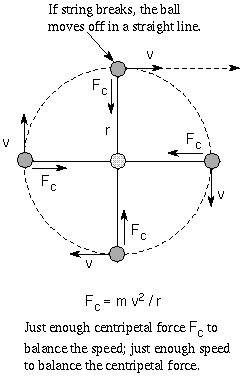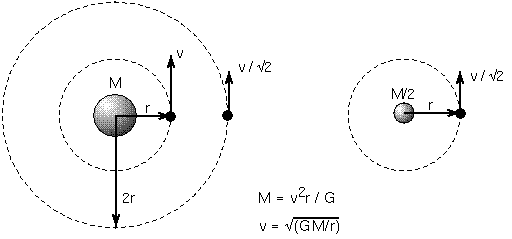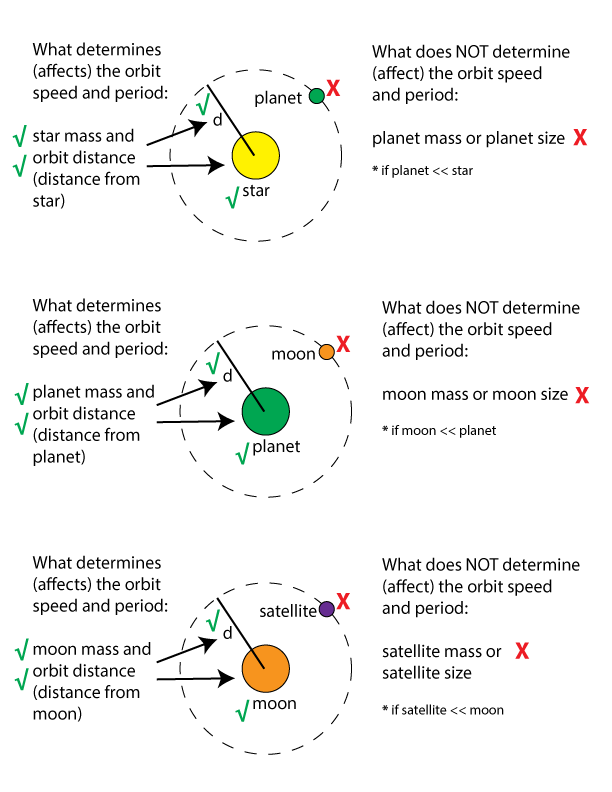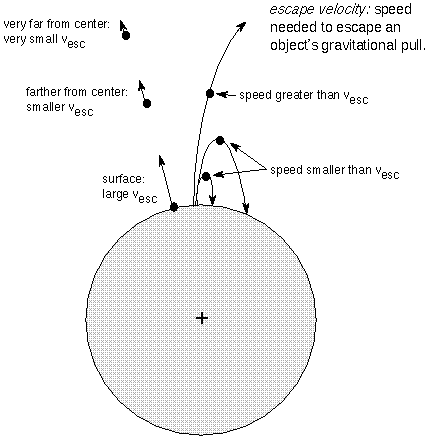## Orbits

#### Chapter index in this window —   — Chapter index in separate window

This material (including images) is copyrighted!. See my copyright notice for fair use practices.

Now I will apply Newton's laws of motion and gravity to topics more astronomical: objects moving around other objects. What kinds of things can you find out about celestial objects from just observing their motions?

### Centripetal ForceNewton's first law of motion says that an object's inertia will keep it from changing its speed and/or direction unless some force acts on it. This means that satellites orbiting the Earth must be feeling some force that constantly deflects them toward the center of the Earth. If there was no force, they would travel in a straight line at a constant speed.

If you whirl a ball attached to string around your head, it moves in a circular path around you because the string is always pulling the ball directly toward the hand grabbing the string. The ball wants to move in a straight line and the string is pulling it directly inward. The resulting deflection is a compromise: a circular path. The string is applying a centripetal force to the ball: an inward force. If you let go of the string, there is no centripetal force and the ball will fly off in a straight line because of its inertia. If you do not whirl the ball fast enough it will move inward to a smaller non-circular path around you. If you whirl the ball too fast, you may not be able to give it enough centripetal force to keep it in a circular path around you. The amount of centripetal force needed to balance an object's inertia and keep it in a circular path of radius r is found from Newton's second law: the centripetal force = m v2 / r, where v and m are the object's speed and mass, respectively. The radius of the orbit r is the same as the distance between the moving object and the central body.

### Measuring Planet and Star Masses

Now for orbits! Satellites are not being deflected by strings but by gravity. Gravity provides the centripetal force needed to keep the satellites in orbit. If you focus on the simple case of circular orbits, you can use the centripetal force formula above with the law of gravity to determine the mass of a planet or star. Simply set the force of gravity equal to the centripetal force and solve for the mass of the planet or star, M.
 (G M m) r2
=
 m v2 r
.
The satellite mass m cancels out from both sides and if you put M on one side and the rest on the other side of the equation, you get
M =
 r2 G
×
 v2 r
M =
 r v2 G
.
This assumes that the satellite's mass, m, is much less than the central object's mass so you can ignore the acceleration of the central object toward the satellite!

### How do you do that?

Let's use this result to get an estimate of the mass of the Sun. You need to use something orbiting with a known radius and speed. The Earth's orbit is roughly circular with radius = 1.5 × 1011 meters and the Earth moves with a speed 30,000 meters/second (= 30 km/s) in its orbit. The distance is given in meters to match the units of the speed. The distance unit of a meter is used because you will be using the gravitational constant G in your calculation and it uses the meter unit. When you do a calculation, you must be sure you check that your units match up or you will get nonsense answers.

Plug the values into the mass relation:
the Sun's mass = (30,000)2 × (1.5 × 1011)/(6.7 × 10-11) = 2 × 1030 kilograms. This is much larger than the Earth's mass so it was okay to ignore the Sun's movement toward the Earth. Using no approximations (ie., not assuming a circular orbit and including the Sun's motion toward the Earth) gives a value for the Sun's mass that is very close to this. Your answer does not depend on which planet you choose to use (here you used the Earth's orbit). You would get the same value for the mass of the Sun if you had used any of the other planets orbital speeds and sizes.

This relation tells you what you need to know in order to measure a planet's or star's mass: the orbital speed of a satellite and the distance it is from the center of the planet or star. Because the velocity is on top of the fraction, satellites are made to move faster if the mass of the central object is greater. At the same distance, a massive planet will exert more gravity force than a low-mass planet, so the massive planet will produce greater inward accelerations on satellites orbiting it. The satellites will, therefore, orbit at faster speeds.

Sometimes the orbital period P is measured instead of the orbital velocity. The orbital period is the time it takes the object to travel the circumference of its orbit (for a circle, the circumference = 2pr, where p is approximately 3.1416). Recall that speed = (distance travelled)/time, so the speed v = the circumference of the orbit/orbital period. When you substitute this for the speed in the mass relation above, you get

M =
 4p2 G
×
 r3 P2
This may look familiar to you---it is Kepler's third law! There is a distance cubed, an orbital period squared, and some other constant factors. Newton found that when Kepler used the motions of the planets to formulate his third law, Kepler was actually measuring the mass of the Sun. If you use the convenient set of units of an astronomical unit for the distance, a year for the time, and a ``solar mass'' (mass relative to the Sun) for the mass, the complicated term (4p2)/G becomes the simple value of 1. For the planets orbiting the Sun, the mass relation becomes 1 = r3 / P2, or r3 = P2, just what Kepler found.### Orbital Speed

The mass formula above tells you that satellites orbiting massive planets must move faster than satellites orbiting low-mass planets at the same distance. Massive planets have stronger gravity than low-mass planets so a satellite orbiting a massive planet is accelerated by a greater amount than one going around a lesser mass planet at the same distance. To balance the stronger inward gravitational pull of the massive planet, the satellite must move faster in its orbit than if it was orbiting a lesser mass planet. Of course, this also applies to planets orbiting stars, stars orbiting other stars, etc.

If you solve for the orbit speed, v, in the mass formula, you can find how fast something needs to move to balance the inward pull of gravity:

v2 = (G M)/r .
Taking the square root of both sides (you want just v not v2), you get
v = Sqrt[(G M)/r].

### How do you do that?

Find the orbital speed of Jupiter around the Sun. Jupiter's distance from the Sun is 5.2 A.U., or 7.8×1011 meters and the Sun's mass is 2×1030 kilograms. The orbital speed of Jupiter around the Sun is Sqrt[6.7×10-11 × (2×1030)/(7.8×1011)] = Sqrt[1.718×108] = 1.3×104 meters/second, or 13 kilometers/second. What do you think you wouldd find if you used one of the Trojan asteroids, millions of times less massive than Jupiter, that orbits the Sun at 5.2 A.U.?

Notice that the orbital speed v (and therefore, the orbital period P) does not depend on the mass of the satellite! The mass M in the formula above is the mass of the central object. If we consider the Sun-Jupiter-Trojan asteroid example in the "How do you do that?" box above (and recall the discussion in the Gravitational Acceleration section), the Sun-Jupiter gravity force is MUCH greater than the Sun-Trojan asteroid gravity force but Jupiter has much greater inertia (resistance to change = mass). The greater gravity force between the Sun and Jupiter is compensated for by the greater inertia of Jupiter so the acceleration of Jupiter will be the same as the acceleration of the Trojan asteroid---Jupiter and the Trojan asteroid will have the same orbital velocity and same orbital period.### Escape Velocity

If an object moves fast enough it can escape a massive object's gravity and not be drawn back toward the massive object. The critical speed needed to do this is the escape velocity. More specifically, this is the initial speed something needs to escape the object's gravity and assumes that there is no other force acting on the object besides gravity after the initial boost. Rockets leaving the Earth do not have the escape velocity at the beginning but the engines provide thrust for an extended period of time, so the rockets can eventually escape. The concept of escape velocity applies to anything gravitationally attracted to anything else (gas particles in planet atmospheres, comets orbiting the Sun, light trying to escape from black holes, galaxies orbiting each other, etc.).Using Newton's laws of motion and law of gravity, you can find that the escape velocity vesc looks very similar to the orbital speed:

vesc = Sqrt[(2 G M)/r].
This is a factor Sqrt larger than the circular orbital speed. Since the mass M is on top of the fraction, the escape velocity increases as the mass increases. More massive bodies exert greater gravity force, so escaping objects have to move faster to overcome the greater gravity. Also, the distance from the center of the object r is in the bottom of the fraction, so the escape velocity DEcreases as the distance increases. Gravity decreases with greater distance, so objects farther from a massive body do not need to move as quickly to escape it than those closer to it.

### How do you do that?

Find the escape velocity from the surface of the Earth. Using the acceleration of gravity, you can find that the Earth has a mass of 6.0×1024 kilograms. The Earth's radius is 6.4×106 meters. Since the mass and distance from the center are in the standard units, you just need to plug their values into the escape velocity relation.

The Earth's surface escape velocity is Sqrt[2× (6.7×10-11) × (6.0×1024)/ (6.4×106)] = Sqrt[1.256×108] = 1.1×104 meters/second (= 11 km/s). Here are some other surface escape velocities: Moon = 2.4 km/s, Jupiter = 59.6 km/s, Sun = 618 km/s.

### Vocabulary

centripetal force escape velocity

### Formulae

1. Mass of central object = [(orbital speed)2 × distance)/G.
2. Mass of central object (Kepler's 3rd law) = (4p2)/G × [(distance)3/(orbital period)2].
3. Orbital speed = Sqrt[G × Mass / distance].
4. Escape velocity = Sqrt[2G × Mass / distance].

### Review Questions

1. What keeps satellites orbiting the Earth moving along their curved paths?
2. What two things must be determined first in order to calculate the mass of a planet or a star?
3. Jupiter's moon Io has about the same mass as the Moon and orbits Jupiter at about the same distance that the Moon orbits the Earth (center to center). Then why does Io take only 1.8 days to orbit Jupiter but our Moon takes 27.3 days to orbit the Earth?
4. Astronomers were able to accurately measure the orbital periods of the moons of Jupiter since the time of Galileo, so why was an accurate value for Jupiter's mass not found for over 300 years until the astronomical unit was measured accurately?
5. Which would have a shorter orbital period, a planet orbiting a massive star at 3 A.U. or a planet orbiting a low-mass star at 3 A.U.? Explain your answer.
6. If a planet orbiting a massive star has the same orbital period as a planet orbiting a low-mass star, which of the planets orbits at a greater distance from its star? Explain your answer.
7. What two things does the escape velocity depend on?
8. Why does the planet Saturn with over 95 times the Earth's mass have a smaller escape velocity at its cloudtops than the Earth has at its cloudtops?
9. Why is Jupiter's escape velocity at its cloudtops over two times higher than the Earth's surface escape velocity, even though Jupiter has a much larger diameter than the Earth?

#### Go to Astronomy Notes home

last updated: May 20, 2019